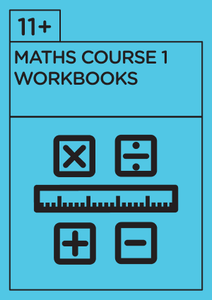Limited Availability - FREE UK Delivery# 11+ Mathematics - Revision Course 1 - Workbooks

Regular price
£95.00
Sale price
£95.00
Regular price
Sold out
Unit price
per
Tax included.

##### 4 Workbooks included, covering:
• Number
• Place value, ordering
• Calculation (addition, subtraction, multiplication & division)
• Remainders
• Mixed computations
• Missing number/symbol problems
• Fractions (addition, subtraction, equivalent, simplifying, multiplication, word problems)
• Decimals, percentages & ratio
• HCF & LCM
• Time, distance, speed
• Estimating/rounding
• Number sequences & patterns
• Shape & measurement (perimeter, area and volume)
• Symmetry
• Data
• Time
• Problem solving
• Maths Test

Answers are also included.

Total Length: 184 pages